# The Gram-Schmidt Process and Orthogonal Vectors

Suppose I gave you some red fingerpaint and asked you to make all the colors you could from this paint. You’d probably come up with a diverse collection of pinks, reds and burgendys – going through the range of reds – but you would be unable to produce a color that does not depend solely on red, like purple.If I were to ask you to produce purple, your reply may be something like “well, give me blue and I’ll be able to color in purple”. When we think in terms of colors, it is easy to understand the concept of the span of a set of colors. In this context, span refers to the set of colors we can create from our original colors.

Now, lets think in terms of numbers (actually vectors) instead of colors. If I gave you a similar task as above, but instead of the color red, I gave you the vector (1, 0, 0) and told you to see what other numbers you could get from this (by scalar multiplication and the addition of any two vectors already produced) then you would probably come back to me and show me how you could use the vector (1, 0, 0) to produce the entire real number line in that first dimension, but leaving the other coordinates at 0.

If (similar to the color example) I asked you to use the vector (1, 0, 0) to produce the vector (1, 1, 0), then you may reply with a similar statement as above: “you give me the vector (0, 1, 0) and I’ll produce (1, 1, 0)”. That’s because the vectors (1, 0, 0) and (1, 1, 0) are linearly independent. This is a mathematical way of saying what we’ve already stated, that you cannot get one vector as a scalar multiple of the other vector for any real number scalar.

If two vectors are linearly independent then neither one belongs to the span of the other. Just as you cannot create blue from red, you cannot create red from blue. So this means that if I were to give you the colors red and blue, then the set of colors that you can create has increased from what you could create from either only red or only blue. Similarly, the vector sets {(1, 0, 0), (0, 1, 0)} spans more vectors than just {(1, 0, 0)} or {(0, 1, 0)}.

Consider then the following two sets of vectors: {(1, 0, 0), (0, 1, 0)} and {(1, 0, 0), (1, 1, 0)}. Notice that (1, 1, 0) [belongs to] span({(1, 0, 0), (0, 1, 0)}) because (1, 1, 0) = (1, 0, 0) + (0, 1, 0). Likewise (0, 1, 0) [belongs to] span({(1, 0, 0), (1, 1, 0) because (0, 1, 0) = (1, 1, 0) – (1, 0, 0). So we can see that these two sets span the same sets of vectors. But which is a better set to use?

Lets to back to colors and think of the set {red, purple}. Here we can think of purple as a simple combination of red and blue (i.e. purple = red + blue). What happens if we mix red and purple? If we think of it in terms of an axis, the fact that purple contains red in it means that as we walk along the purple axis, we are walking along the red axis as well. If we considered the set {red, blue}, we see that this is not happening. As we change the amount of red in our color, the amount of blue is unaffected. Likewise, if we change the amount of blue, the amount of red is not affected. If we were to draw these axes, we could see that this happens because the colors red and blue are perpendicular (or orthogonal) to one another, while red and purple are not.Replacing these colors with vectors again, we get the same thing. We have the option of using the vector set {(1, 0, 0), (1, 1, 0)} or {(1, 0, 0), (0, 1, 0)} as our axis and it is better to use the second set because the two vectors are not just independent of one another, but also are at a 90[degree] angle, or are orthogonal to one another.

When given a set of vectors (or a set of colors), an important problem is to determine the span of those vectors and to be able to produce an orthogonal set of vectors that spans that same space. The Gram-Schmidt process provides a procedure for producing these orthogonal vectors.

The way the procedure works is to build an orthogonal set of vectors from the original set by computing the projection of the current vector being worked on in terms of the previous vectors in the orthogonal set. This projection procedure is defined as proju(v) = (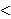u, v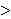/u, u)u. The formula for the ith vector of the Gram-Schmidt process is

ui = vi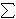j = 1 to i-1 projuj(vi).

Here is a script I’ve written to help with this process.

# Permutation Problems

I love to play with puzzles. When I was in grade school I would spend hours at a time figuring out ways to solve from things like Tetris, Mindsweeper, Solitare, and Freecell. Later I was introduced to puzzles involving numbers like Sudoku and Nonograms.

These puzzles are often interesting in part because there is generally a very large way that things can be arranged, but only a few of these arrangements are correct. Generally a person solving a puzzle will figure out certain things that must be true or cannot be true, which helps in solving the puzzle and reducing the number of possible cases. Initially, though, we are often left with a situation where we have a new puzzle and our only method is to keep trying every possible solution until we start to notice a pattern (or reach a solution).

For example, consider a Sudoku puzzle. We are given a partially filled in grid and our job is to fill in the remaining cells with the rules that every row, column and marked subgrid must have the numbers 1 – 9 exactly once. One initial attempt at solving such a puzzle could be to attempt to permute the string 1, 2, 3, 4, 5, 6, 7, 8, 9 until we find a solution that fits the first row, then do the same with the second row, and so on and so forth.

One immediate question is how many ways are there to permute the numbers 1, …, 9? We can answer this by realizing that each permutation is a new string. So for each string that we construct, we have 9 choices for the first element in the string. Then once that element has been chosen, we are not allowed for that element to appear anywhere else. So there are only 8 possible choices for what can go in the second string. Continuing this process, we see that the number of possible permutations we can construct from the string 1, …, 9 is

9 * 8 * 7 * 6 * 5 * 4 * 3 * 2 * 1 = 362880

This is a large number of possible strings to generate just to get one row of a Sudoku, so hopefully you’ll be able to notice the pattern before going through this whole set (because once you’ve generated the first row you still have to do the other 8 rows).

Nonetheless because there is often great value that can be gained by knowing how to permute through all possible solutions, I have written three functions that help with this process: Next_Permutation, Previous_Permutation, and Random_Permutation.

Before I give these algorithms, I want to highlight two notations on ordering string. A string (a1, a2, …, an) is said to be in lexicographical order (or alphabetical order) if for each i [in] 1, …, n-1, ai [<=] ai+1. Likewise, a string is said to be in reverse lexicographical order if for each i [in] 1, …, n-1, ai [>=] ai+1.

Next Permutation

If the given string is not in reverse lexicographic order, then there exists two elements j1 and j2 such that j1 [<] j2 and aj1 [<] aj2.
1. The Next_Permutation algorithm first searches for the largest element j1 such that aj1 [<] aj1 + 1. Since we said the string is not in reverse lexicographic order, this j1 must exist.
2. Once this j1 is found, we search for the smallest element aj2 such that aj2 [>] aj1. Again, since we know that this is true for j1 + 1, we know that such a j2 must exist.
3. We swap the elements aj1 and aj2.
4. The elements after j1 + 1 are then placed in lexicographic order (i.e. in order such that ai [>=] ai+1

If the given string is in reverse lexicographic order, then we simply reverse the string.

Previous Permutation

If the given string is not in lexicographic order, then there exists two elements j1 and j2 such that j1 [<] j2 and aj1 [>] aj2.
1. The Previous_Permutation algorithm first searches for the largest element j1 such that aj1 [>] aj1 + 1. Since we said the string is not in reverse lexicographic order, this j1 must exist.
2. Once this j1 is found, we search for the smallest element aj2 such that aj1 [>] aj2. Again, since we know that this is true for j1 + 1, we know that such a j2 must exist.
3. We swap the elements aj1 and aj2.
4. The elements after j1 + 1 are then placed in reverse lexicographic order (i.e. in order such that ai [<=] ai+1

If the given string is in lexicographic order, then we simply reverse the string.

Random Permutation

This generates a random string permutation.

This script can be seen here, set to work on permutations of colors.

# My Review of “The Golden Ticket: P, NP, and the Search for the Impossible”I came home from work on Wednesday a bit too tired to go for a run and a bit too energetic to sit and watch TV. So I decided to pace around my place while reading a good book. The question was did I have a good book to read. I had been reading sci-fi type books earlier this month and wanted a break from that, so I looked in my mailbox and noticed that I had just received my copy of “The Golden Ticket: P, NP, and the Search for the Impossible“. At the time, I was of the mindset that I had just gotten off of work and really didn’t want to be reading a text book as if I was still at work. But I decided to give it a try and at least make it through the first few pages and if it got to be overwhelming, I’d just put it down and do something else.

About three hours later I was finishing the final pages of the book and impressed that the author (Lance Fortnow) was able to treat complexity theory the same way that I see physics professors speaking about quantum physics and the expanding universe on shows like “the Universe” and “Through the Wormhole with Morgan Freeman” where complex topics are spoken about with everyday terminology. It wouldn’t surprise me to see Dr. Fortnow on shows like “The Colbert Report” or “The Daily Show” introducing the topics in this book to a wider audience.

Below is the review I left on Amazon.

`I really enjoyed this book. It was a light enough read to finish in one sitting on a weeknight within a few hours, but also showed its importance by being able to connect the dots between the P = NP problem to issues in health care, economics, security, scheduling and a number of other problems. And instead of talking in a "professor-like" tone, the author creates illustrative examples in Chapters 2 and 3 that are easy to grasp. These examples form the basis for much of the problems addressed in the book.`

`This is a book that needed to be written and needs to be on everyone's bookshelf, particularly for those asking questions like "what is mathematics" or "what is mathematics used for". This book answers those questions, and towards the end gives examples (in plain English) of the different branches of mathematics and theoretical computer science, without making it read like a text book. `

# Approximating the Set Cover ProblemI just finished my weekly task of shopping for groceries. This can be a somewhat daunting task because I generally have a list of things that I’ll need which cannot all be purchased at a single location. What often happens is that I find that many of the items on my list are ONLY offered at certain stores – generic brands of certain items for example. My goal then changes from minimizing the total amount of money spent to minimizing the number of stores that I must visit to purchase all of my items.

To formulate this as a mathematical problem, suppose that I have a grocery list of items I would like to buy, represented by the lists item1, item2, …, itemn, where n represents the number of items I have on this list. Suppose also that there are stores Store1, Store2, …, Storem (each one distinct) that offer some combination of items I have on my list. What I would like to do is minimize the number of stores I have to visit to purchase these items.

The problem I just described is famous because it is one that many people face on a regular basis. In a more general form, it is so famous that it has a name for it, called the Set Cover Problem (or the Minimum Set Cover Problem). In the general form of this problem, we replace the grocery list with a set of items called our universe. The lists of items offered at each store are the collections of subsets of the universe. In the problem, as in the example above, we would like to select enough subsets from this collection that we are able to obtain every element in our universe. We would like to do this with as low a number of sets as possible.

In my previous post, I described the 21 problems that Karp proved were NP-Complete. Set Cover was one of those problems, showing that this is a hard problem to solve. What I will do is introduce three ways to reach a near-optimal solution relatively quickly.

Greedy Method

One of the first approaches one may take to solve this problem is to repeatedly select the subset that contains the most new items. That’s how the greedy approach to set cover operates. The method knows to terminate when all elements belong to one of the selected sets. In the shopping example above, this would be accomplished by visiting the store that had the most items on my list and purchasing those items at this store. Once this is done, the items that have been purchased can be crossed off my list and we can visit the store with the most items on my remaining list, stopping when the list is empty.

Linear Programming Relaxation

Instead of stating the set cover problem with words, there is a way of describing the situation with mathematical inequalities. For instance, suppose that the soap I like to purchase is only available at stores Store1, Store4 and Store9. Then I could introduce a variable xi for each store i and the requirement that I purchase this soap can be restated as :

x1 + x4 + x9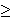1

Because we can either purchase some items or not purchase these items, each variable xi is 0 or 1 (called a binary variable). We can introduce similar constraints for each element in our universe (or on our grocery list). These inequalities (called constraints) have the form:

for each element e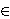U,i | eSi xi1

Our goal of minimizing the number of sets chosen (stores visited) can be stated by the objective function:
minimize1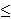in xi

So the mathematical formulation for this problem can be stated as

minimize1in xi
Subject to
for each element eU,i | eSi xi1
for each set i, xi{0, 1}.

Formulations of this type, where variables are restricted to a finite set (in this case the x variables being either 0 or 1) are called integer programs. Unfortunately, there is no easy way to solve these formulations either. However, there is a related problem which can be solved quickly.

Instead of restricting the x variables to the values of 0 or 1, we could allow them to take on any value within this range, i.e. 0xi1 for each set Si. Doing this converts the problem from an integer programming problem into a linear programming problem (called the LP-Relaxation), which can be solved quickly. The issue with this method though is that the solution obtained by an LP-Relaxation is not guaranteed to be an integer. In this case, how do we interpret the values xi?

Randomized Rounding Method

One approach to dealing with a non-integer solution to the LP-Relaxation is to treat the xi values as probabilities. We can say that xi is the probability that we select set i. This works because each value of xi is in the range of 0 to 1, which is necessary for a probability. We need to repeatedly select sets with their associated probabilities until all elements in our universe are covered. Selecting our sets based on this procedure is the randomized rounding approach.

Deterministic Rounding Method

A second approach to dealing with a non-integer solution to the LP-Relaxation is to base our solution on the most occurring element. If we let f be this frequency (i.e.the number of sets that the most occurring element occurs in), then we can define a solution by selecting set i if the LP=Relaxation solution gives the variable xi a value of at least (1/f).

None of these three approaches is guaranteed to give an optimal solution to an instance of this problem. I will not go into it in this post, but these can all be shown to be within some guaranteed range of the optimal solution, thus making them approximation algorithms.

Hope you enjoy.